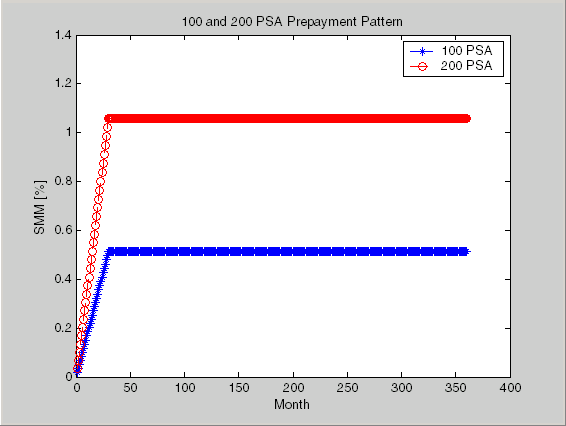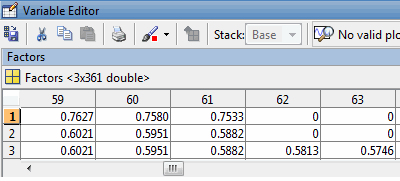## Fixed-Rate Mortgage Pool

### Introduction

Financial Instruments Toolbox™ software supports calculations involved with generic fixed-rate mortgage pools and balloon mortgages. Generic fixed-rate mortgage pools and balloon mortgages have pass-through certificates (PC) that typically have embedded call options in the form of prepayment. Prepayment is an excess payment applied to the principal of a PC. These accelerated payments reduce the effective life of a PC.

The toolbox comes with a standard Bond Market Association (PSA) prepayment model and can generate multiples of standard prepayment speeds. The Public Securities Association provides a set of uniform practices for calculating the characteristics of mortgage-backed securities when there is an assumed prepayment function.

Alternatively, aside from the standard PSA implementation in this toolbox, you can supply your own projected prepayment vectors. Currently, however, custom prepayment functionality that incorporates pool-specific information and interest rate forecasts are not available in this toolbox. If you plan to use custom prepayment vectors in your calculations, you presumably already own such a suite in MATLAB®.

### Inputs to Functions

Because of the generic, all-purpose nature of the toolbox pass-through functions, you can fine-tune them to conform to a particular mortgage. Most functions require at least this set of inputs:

• Gross coupon rate

• Settlement date

• Issue (effective) date

• Maturity date

Typical optional inputs include standard prepayment speed (or customized vector), net coupon rate (if different from gross coupon rate), and payment delay in number of days.

All calculations are based on expected payment dates and actual cash flow to the investor. For example, when `GrossRate` and `CouponRate` differ as inputs to `mbsdurp`, the function returns a modified duration based on `CouponRate`. (A notable exception is `mbspassthrough`, which returns interest quantities based on the `GrossRate`.)

### Generating Prepayment Vectors

You can generate PSA multiple prepayment vectors quickly. To generate prepayment vectors of 100 and 200 PSA, type

```PSASpeed = [100, 200]; [CPR, SMM] = psaspeed2rate(PSASpeed); ```

This function computes two prepayment values: conditional prepayment rate (CPR) and single monthly mortality (SMM) rate. CPR is the percentage of outstanding principal prepaid in one year. SMM is the percentage of outstanding principal prepaid in one month. In other words, CPR is an annual version of SMM.

Since the entire 360-by-2 array is too long to show in this document, observe the SMM (100 and 200 PSA) plots, spaced one month apart, instead.Prepayment assumptions form the basis upon which far more comprehensive MBS calculations are based. As an illustration, observe the following example, which shows the use of the function `mbscfamounts` to generate cash flows and timings based on a set of standard prepayments.

Consider three mortgage pools that were sold on the issue date (which starts unamortized). The first two pools "balloon out" in 60 months, and the third is regularly amortized to the end. The prepayment speeds are assumed to be 100, 200, and 200 PSA, respectively.

```Settle = [datenum('1-Feb-2000'); datenum('1-Feb-2000'); datenum('1-Feb-2000')]; Maturity = [datenum('1-Feb-2030')]; IssueDate = datenum('1-Feb-2000'); GrossRate = 0.08125; CouponRate = 0.075; Delay = 14; PSASpeed = [100, 200]; [CPR, SMM] = psaspeed2rate(PSASpeed); PrepayMatrix = ones(360,3); PrepayMatrix(1:60,1:2) = SMM(1:60,1:2); PrepayMatrix(:,3) = SMM(:,2); [CFlowAmounts, CFlowDates, TFactors, Factors] = ... mbscfamounts(Settle, Maturity, IssueDate, GrossRate, ... CouponRate, Delay, [], PrepayMatrix); ```

The fourth output argument, `Factors`, indicates the fraction of the balance still outstanding at the beginning of each month. A snapshot of this argument in the MATLAB Variables editor illustrates the 60-month life of the first two of the mortgages with balloon payments and the continuation of the third mortgage until the end (360 months).You can readily see that `mbscfamounts` is the building block of most fixed rate and balloon pool cash flows.

### Mortgage Prepayments

Prepayment is beneficial to the pass-through owner when a mortgage pool has been purchased at discount. The next example compares mortgage yields (compounded monthly) versus the purchase clean price with constant prepayment speed. The example illustrates that when you have purchased a pool at a discount, prepayment generates a higher yield with decreasing purchase price.

```Price = [85; 90; 95]; Settle = datenum('15-Apr-2002'); Maturity = datenum('1 Jan 2030'); IssueDate = datenum('1-Jan-2000'); GrossRate = 0.08125; CouponRate = 0.075; Delay = 14; Speed = 100; ```

Compute the mortgage and bond-equivalent yields.

```[MYield, BEMBSYield] = mbsyield(Price, Settle, Maturity, ... IssueDate, GrossRate, CouponRate, Delay, Speed)```
```MYield = 0.1018 0.0918 0.0828 BEMBSYield = 0.1040 0.0936 0.0842 ```

If for this same pool of mortgages, there was no prepayment (`Speed = 0`), the yields would decline to

```MYield = 0.0926 0.0861 0.0802 BEMBSYield = 0.0944 0.0877 0.0815 ```

Likewise, if the rate of prepayment doubled (```Speed = 200```), the yields would increase to

```MYield = 0.1124 0.0984 0.0858 BEMBSYield = 0.1151 0.1004 0.0873 ```

For the same prepayment vector, deeper discount pools earn higher yields. For more information, see `mbsprice` and `mbsyield`.

### Risk Measurement

Financial Instruments Toolbox provides the most basic risk measures of a pool portfolio:

• Modified duration

• Convexity

• Average life of pool

Consider the following example, which calculates the Macaulay and modified durations given the price of a mortgage pool.

```Price = [95; 100; 105]; Settle = datenum('15-Apr-2002'); Maturity = datenum('1-Jan-2030'); IssueDate = datenum('1-Jan-2000'); GrossRate = 0.08125; CouponRate = 0.075; Delay = 14; Speed = 100; [YearDuration, ModDuration] = mbsdurp(Price, Settle, ... Maturity, IssueDate, GrossRate, CouponRate, Delay, Speed)```
```YearDuration = 6.1341 6.3882 6.6339 ModDuration = 5.8863 6.1552 6.4159 ```

Using Financial Instruments Toolbox functions, you can obtain modified duration and convexity from either price or yield, as long as you specify a prepayment vector or an assumed prepayment speed. The toolbox risk-measurement functions (`mbsdurp`, `mbsdury`, `mbsconvp`, `mbsconvy`, and `mbswal`) adhere to the guidelines listed in the PSA Uniform Practices manual.

### Mortgage Pool Valuation

For accurate valuation of a mortgage pool, you must generate interest-rate paths and use them with mortgage pool characteristics to properly value the pool. A widely used methodology is the option-adjusted spread (OAS). OAS measures the yield spread that is not directly attributable to the characteristics of a fixed-income investment.

#### Calculating OAS

Prepayment alters the cash flows of an otherwise regularly amortizing mortgage pool. A comprehensive option-adjusted spread calculation typically begins with the generation of a set of paths of spot rates to predict prepayment. A path is collection of i spot-rate paths, with corresponding j cash flows on each of those paths.

The effect of the OAS on pool pricing is shown mathematically in the following equation, where K is the option-adjusted spread.

`$PoolPrice=\frac{1}{NumberofPaths}×\sum _{i}^{NumberofPaths}\sum _{j}^{C{F}_{ij}}\frac{C{F}_{ij}}{{\left(1+zerorate{s}_{ij}+K\right)}^{T}{}^{{}_{ij}}}$`

#### Calculating Effective Duration

Alternatively, if you are more interested in the sensitivity of a mortgage pool to interest rate changes, use effective duration, which is a more appropriate measure. Effective duration is defined mathematically with the following equation.

`$Effective\text{\hspace{0.17em}}\text{\hspace{0.17em}}Duration=\frac{P\left(y+\Delta y\right)-P\left(y-\Delta y\right)}{2P\left(y\right)\Delta y}$`

#### Calculating Market Price

The toolbox has all the components required to calculate OAS and effective duration if you supply prepayment vectors or assumptions. For OAS, given a prepayment vector, you can generate a set of cash flows with `mbscfamounts`. Discounting these cash flows with the reference curve and then adding OAS produces the market price. See Computing Option-Adjusted Spread for a discussion on the computation of option-adjusted spread.

Effective duration is a more difficult issue. While modified duration changes the discounting process (by changing the yield used to discount cash flows), effective duration must account for the change in cash flow because of the change in yield. A possible solution is to recompute prices using `mbsprice` for a small change in yield, in both the upwards and downwards directions. In this case, you must recompute the prepayment input. Internally, this alters the cash flows of the mortgage pool. Assuming that the OAS stays constant in all yield environments, you can apply a set of discounting factors to the cash flows in up and down yield environments to find the effective duration.